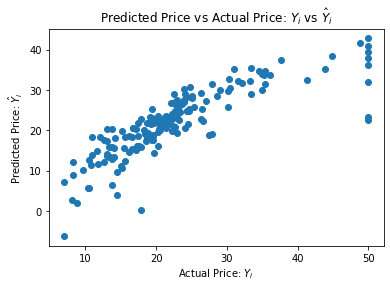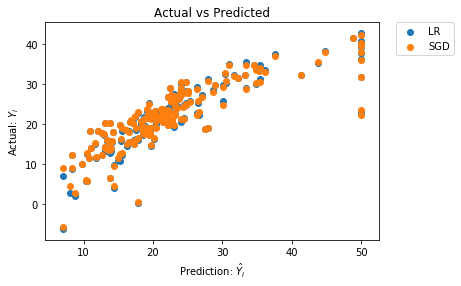# Stochastic Gradient Descent (SGD) Regression¶

## A Basic Example Using the Boston House Prices Dataset¶

### Overview¶

Linear regression (i.e. ordinary least squares) is one of the most commonly used statistical modeling technique. In this example, we execute a linear regression model (lr) and then compare it to a Stochastic Gradient Descent (sgd) method. We will not go into the mathematical details of either model. A few resources are listed below if you are interested in a deeper dive.

### Data Exploration¶

First we import a the boston house prices dataset, and print a description of it so we can examine what is in the data. Remember in order to execute a 'cell' like the one below, you can 1) click on it and run it using the run button above or 2) click in the cell and hit shift+enter.

In :
import pandas as pd
print(boston.data.shape) #get (numer of rows, number of columns or 'features')
print(boston.DESCR) #get a description of the dataset

(506, 13)
.. _boston_dataset:

Boston house prices dataset
---------------------------

**Data Set Characteristics:**

:Number of Instances: 506

:Number of Attributes: 13 numeric/categorical predictive. Median Value (attribute 14) is usually the target.

:Attribute Information (in order):
- CRIM     per capita crime rate by town
- ZN       proportion of residential land zoned for lots over 25,000 sq.ft.
- INDUS    proportion of non-retail business acres per town
- CHAS     Charles River dummy variable (= 1 if tract bounds river; 0 otherwise)
- NOX      nitric oxides concentration (parts per 10 million)
- RM       average number of rooms per dwelling
- AGE      proportion of owner-occupied units built prior to 1940
- DIS      weighted distances to five Boston employment centres
- TAX      full-value property-tax rate per $10,000 - PTRATIO pupil-teacher ratio by town - B 1000(Bk - 0.63)^2 where Bk is the proportion of blacks by town - LSTAT % lower status of the population - MEDV Median value of owner-occupied homes in$1000's

:Missing Attribute Values: None

:Creator: Harrison, D. and Rubinfeld, D.L.

This is a copy of UCI ML housing dataset.
https://archive.ics.uci.edu/ml/machine-learning-databases/housing/

This dataset was taken from the StatLib library which is maintained at Carnegie Mellon University.

The Boston house-price data of Harrison, D. and Rubinfeld, D.L. 'Hedonic
prices and the demand for clean air', J. Environ. Economics & Management,
vol.5, 81-102, 1978.   Used in Belsley, Kuh & Welsch, 'Regression diagnostics
...', Wiley, 1980.   N.B. Various transformations are used in the table on
pages 244-261 of the latter.

The Boston house-price data has been used in many machine learning papers that address regression
problems.

.. topic:: References

- Belsley, Kuh & Welsch, 'Regression diagnostics: Identifying Influential Data and Sources of Collinearity', Wiley, 1980. 244-261.
- Quinlan,R. (1993). Combining Instance-Based and Model-Based Learning. In Proceedings on the Tenth International Conference of Machine Learning, 236-243, University of Massachusetts, Amherst. Morgan Kaufmann.


In :
data = pd.DataFrame(boston.data, columns=boston.feature_names)

Out:
CRIM ZN INDUS CHAS NOX RM AGE DIS RAD TAX PTRATIO B LSTAT
0 0.00632 18.0 2.31 0.0 0.538 6.575 65.2 4.0900 1.0 296.0 15.3 396.90 4.98
1 0.02731 0.0 7.07 0.0 0.469 6.421 78.9 4.9671 2.0 242.0 17.8 396.90 9.14
2 0.02729 0.0 7.07 0.0 0.469 7.185 61.1 4.9671 2.0 242.0 17.8 392.83 4.03
3 0.03237 0.0 2.18 0.0 0.458 6.998 45.8 6.0622 3.0 222.0 18.7 394.63 2.94
4 0.06905 0.0 2.18 0.0 0.458 7.147 54.2 6.0622 3.0 222.0 18.7 396.90 5.33
In :
#For some reason, the loaded data does not include the target variable (MEDV), we add it here
data['MEDV'] = pd.Series(data=boston.target, index=data.index)
data.describe() #get some basic stats on the dataset

Out:
CRIM ZN INDUS CHAS NOX RM AGE DIS RAD TAX PTRATIO B LSTAT MEDV
count 506.000000 506.000000 506.000000 506.000000 506.000000 506.000000 506.000000 506.000000 506.000000 506.000000 506.000000 506.000000 506.000000 506.000000
mean 3.613524 11.363636 11.136779 0.069170 0.554695 6.284634 68.574901 3.795043 9.549407 408.237154 18.455534 356.674032 12.653063 22.532806
std 8.601545 23.322453 6.860353 0.253994 0.115878 0.702617 28.148861 2.105710 8.707259 168.537116 2.164946 91.294864 7.141062 9.197104
min 0.006320 0.000000 0.460000 0.000000 0.385000 3.561000 2.900000 1.129600 1.000000 187.000000 12.600000 0.320000 1.730000 5.000000
25% 0.082045 0.000000 5.190000 0.000000 0.449000 5.885500 45.025000 2.100175 4.000000 279.000000 17.400000 375.377500 6.950000 17.025000
50% 0.256510 0.000000 9.690000 0.000000 0.538000 6.208500 77.500000 3.207450 5.000000 330.000000 19.050000 391.440000 11.360000 21.200000
75% 3.677083 12.500000 18.100000 0.000000 0.624000 6.623500 94.075000 5.188425 24.000000 666.000000 20.200000 396.225000 16.955000 25.000000
max 88.976200 100.000000 27.740000 1.000000 0.871000 8.780000 100.000000 12.126500 24.000000 711.000000 22.000000 396.900000 37.970000 50.000000
In :
#Load the independent variables (the x1, x2, etc.) into a dataframe object called 'X'. Similarly for the dependent variable 'Y'
X = data.drop('MEDV', axis = 1) #define independent predictor set (excluding the dependent variable)
Y = data['MEDV'] #define the target values (i.e. the dependent variable)


We randomly select a third of our data to be the 'test' dataset. This way we can train our model on 2/3 of the data, and test it on the remainder. Once we are confident that our model is generalizing well (i.e. there is not a HUGE different in the training/testing performance, or in other words, not obviously overfitting), then we can use all of our data to train the model.

In :
from sklearn.model_selection import train_test_split
X_train, X_test, Y_train, Y_test = train_test_split(X, Y, test_size = 0.33, random_state = 5)
print(X_train.shape)
print(X_test.shape)
print(Y_train.shape)
print(Y_test.shape)

(339, 13)
(167, 13)
(339,)
(167,)


### Basic Linear Regression¶

In linear regression we assume that the relationship between the independent variables (X) and the dependent variable (Y) is linear and then go about finding one that minimizes the squared error between the predicted Y and the actual Y.

$${y}_i = \beta_0 + \beta_1 {x}_i + \epsilon_i$$

We now import the LinearRegression method from the sklearn library. Note that the process of creating the model involves the very simple command lm.fit(X,Y). This runs the model and we find the intercept-term, $\beta_0$, and the coefficient $\beta_1$ that minimizes the squared errors.

In :
from sklearn.linear_model import LinearRegression
lm = LinearRegression()
lm.fit(X_train,Y_train)
y_pred_train = lm.predict(X_train) #predictions on training data
y_pred_test = lm.predict(X_test) #predictions on testing data
# We plot predicted Y (y-axis) against actual Y (x-axis). Perfect predictions will lie on the diagonal. We see the diagonal trend, suggesting a 'good' fit
import matplotlib.pyplot as plt
plt.scatter(Y_test,y_pred_test)
plt.xlabel("Actual Price: $Y_i$")
plt.ylabel("Predicted Price: $\hat{Y}_i$")
plt.title("Predicted Price vs Actual Price: $Y_i$ vs $\hat{Y}_i$")
plt.show()In :
#Let's get the coefficients - observe that some are significantly negative
print('Intercept term: ',lm.intercept_) # This gives us the intercept term
print('Coefficients: \n',lm.coef_) # This gives us the coefficients (in the case of this model, just one coefficient)

Intercept term:  32.8589326340861
Coefficients:
[-1.56381297e-01  3.85490972e-02 -2.50629921e-02  7.86439684e-01
-1.29469121e+01  4.00268857e+00 -1.16023395e-02 -1.36828811e+00
3.41756915e-01 -1.35148823e-02 -9.88866034e-01  1.20588215e-02
-4.72644280e-01]


### Stochastic Gradient Descent SGD Regression¶

Stochastic Gradient Descent (or SGD) is an iterative optimization technique that approximates a smooth, differentiable gradient. While calculating the actual gradient requires all of the data, SGD estimates it using a randomly selected subset of the data. The advantages of this algorithm are simplicity, efficiency of computation and ease of implementation. The disadvantages include having a lot of parameters to tune, the sensitivity of the algorithm to the scale or units of the independent variables, and having to iterate potentially many times without really knowing whether the solution you have in the end is a local or global minima for what you are trying to optimize.

In :
import numpy as np
from sklearn.linear_model import SGDRegressor
from sklearn.pipeline import make_pipeline
from sklearn.preprocessing import StandardScaler
n_samples, n_features = 10, 5
rng = np.random.RandomState(0)
sgd = make_pipeline(StandardScaler(),SGDRegressor(max_iter=1000, tol=1e-3)) # Always scale the input. The most convenient way is to use a pipeline.
sgd.fit(X_train, Y_train)

Out:
Pipeline(memory=None,
steps=[('standardscaler',
StandardScaler(copy=True, with_mean=True, with_std=True)),
('sgdregressor',
SGDRegressor(alpha=0.0001, average=False, early_stopping=False,
epsilon=0.1, eta0=0.01, fit_intercept=True,
l1_ratio=0.15, learning_rate='invscaling',
loss='squared_loss', max_iter=1000,
n_iter_no_change=5, penalty='l2', power_t=0.25,
random_state=None, shuffle=True, tol=0.001,
validation_fraction=0.1, verbose=0,
warm_start=False))],
verbose=False)
In :
#Get predictions from SGD on training and on test data
y_pred_train_sgd = sgd.predict(X_train)
y_pred_test_sgd  = sgd.predict(X_test)


We measure the accuracy of the SGD approach using R-squared metric, and compare it to linear regression. The performance of this algorithm on this dataset is very similar to that of linear regression (on both training and testing data).

In :
from sklearn.metrics import mean_squared_error, mean_absolute_error, r2_score
print('R-square, Training, Linear Regression: ', r2_score(Y_train,Y_pred_train)) #R-Squared from linear regression, on the training data
print('R-square, Testing, Linear Regression: ', r2_score(Y_test,Y_pred_test)) #R-Squared from linear regression, on the training data
print('R-square, Training, SGD: ', r2_score(Y_train,y_pred_train_sgd)) #R-Squared from SGD, Training
print('R-square, Training, SGD: ', r2_score(Y_test,y_pred_test_sgd)) #R-Squared from SGD, Testing

R-square, Training, Linear Regression:  0.7551332741779997
R-square, Testing, Linear Regression:  0.6956551656111607
R-square, Training, SGD:  0.7535303105486528
R-square, Training, SGD:  0.6966892599168628

In :
#Plot results from linear model and SGD
plt.scatter(Y_test, y_pred_test, label='LR')
plt.scatter(Y_test, y_pred_test_sgd, label='SGD')
plt.xlabel("Prediction: $\hat{Y}_i$")
plt.ylabel("Actual: $Y_i$")
plt.title("Actual vs Predicted")
plt.legend(bbox_to_anchor=(1.05, 1), loc='upper left', borderaxespad=0.) # Place a legend to the right of this smaller subplot.
plt.show()You can explore the linear regression model further, here: https://predictivemodeler.com/2019/08/19/py-ols-boston-house-prices/

### Feedback¶

If you have ideas on how to improve this post, please let me know: https://predictivemodeler.com/feedback/